Author: Oscar Cronquist Article last updated on February 10, 2022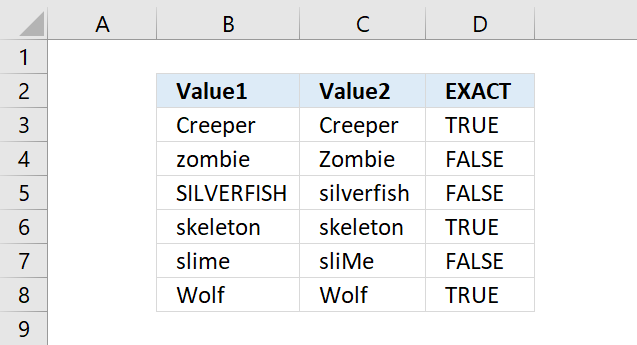The EXACT function allows you to check if two values are precisely the same, it returns TRUE or FALSE. The EXACT function also considers upper case and lower case letters.

Formula in cell D3:

=EXACT(B3,C3)

## 1. Exact Function Syntax

EXACT(text1, text2)

## 2. EXACT Function Arguments

 text1 The first value to compare. Required. text2 The second value to compare. Required.

## 3. Does the EXACT function ignore cell formatting?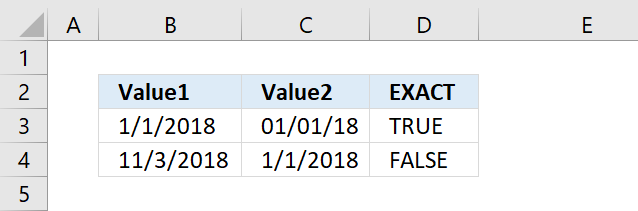Yes, the EXACT function ignores cell formatting differences.

## 4. Case sensitive count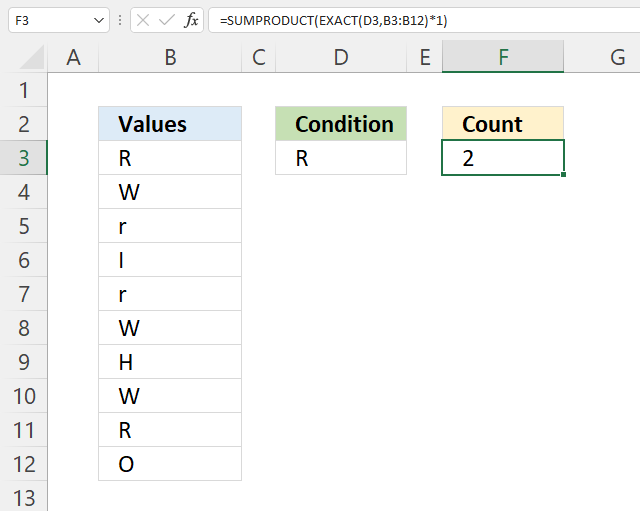The formula in cell F2 counts cells in cell range B3:B12 based on the condition specified in cell D3, the count is case sensitive.

The result is 2, cells B3 and B11 equal the condition in cell D3.

Formula in cell F3:

=SUMPRODUCT(EXACT(D3,B3,B12)*1)

### Explaining formula in cell F3

#### Step 1 - Identify cells equal to condition case sensitive

EXACT(D3,B3,B12)

becomes

EXACT("R",{"R"; "W"; "r"; "I"; "r"; "W"; "H"; "W"; "R"; "O"})

and returns

{TRUE; FALSE; FALSE; FALSE; FALSE; FALSE; FALSE; FALSE; TRUE; FALSE}.

#### Step 2 - Multiply with 1

The SUMPRODUCT function can't add boolean values, we need to convert them to their numerical equivalents.

The asterisk character lets you multiply values in Excel formulas.

EXACT(D3,B3,B12)*1

becomes

{TRUE; FALSE; FALSE; FALSE; FALSE; FALSE; FALSE; FALSE; TRUE; FALSE}*1

and returns

{1; 0; 0; 0; 0; 0; 0; 0; 1; 0}.

#### Step 3 - Sum values

The SUMPRODUCT function adds values and returns a total, the reason we don't use the SUM function is that it requires us to enter the formula as an array formula.

SUMPRODUCT(EXACT(D3,B3,B12)*1)

becomes

SUMPRODUCT({1; 0; 0; 0; 0; 0; 0; 0; 1; 0})

and returns 2.

## 5. Calculate average based on a case sensitive condition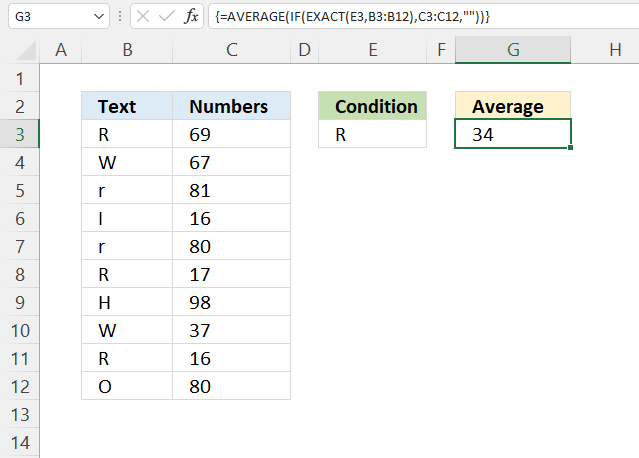Array formula in cell G3:

=AVERAGE(IF(EXACT(E3,B3:B12),C3:C12,""))

### 5.1 How to enter an array formula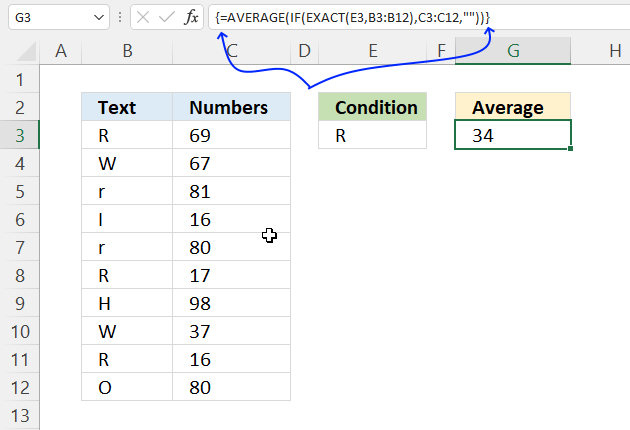The image above shows a leading and trailing curly bracket. They appear automatically when you follow the steps below.

1. Copy the array formula above.
2. Double press with the left mouse button on cell G3, a prompt appears.
3. Paste the formula to cell G3, shortcut keys are CTRL + v.
4. Press and hold CTRL + SHIFT keys simultaneously.
5. Press Enter once.
6. Release all keys.

The formula bar shows a beginning and ending curly bracket, don't enter these characters yourself.

### 5.2 Explaining formula

#### Step 1 - Compare condition to values also considering capital and lower letters

EXACT(E3, B3:B12)

becomes

EXACT("R", {"R"; "W"; "r"; "I"; "r"; "R"; "H"; "W"; "R"; "O"})

and returns

{TRUE; FALSE; FALSE; FALSE; FALSE; TRUE; FALSE; FALSE; TRUE; FALSE}.

#### Step 2 - Replace TRUE with corresponding value on the same row

The IF function returns one value if the logical test is TRUE and another value if the logical test is FALSE.

IF(logical_test, [value_if_true], [value_if_false])

IF(EXACT(E3,B3:B12), C3:C12, "")

beomes

IF({TRUE; FALSE; FALSE; FALSE; FALSE; TRUE; FALSE; FALSE; TRUE; FALSE}, C3:C12, "")

becomes

IF({TRUE; FALSE; FALSE; FALSE; FALSE; TRUE; FALSE; FALSE; TRUE; FALSE}, {69; 67; 81; 16; 80; 17; 98; 37; 16; 80}, "")

and returns

{69; ""; ""; ""; ""; 17; ""; ""; 16; ""}.

#### Step 3 - Calculate the average

The AVERAGE function calculates the average based on a given set of numbers.

AVERAGE(number1[number2], ...)

AVERAGE(IF(EXACT(E3,B3:B12),C3:C12,""))

becomes

AVERAGE({69; ""; ""; ""; ""; 17; ""; ""; 16; ""})

and returns 34. 69 + 17 + 16 = 102. 102/3 = 34.

## 6. Calculate a sum based on a case sensitive condition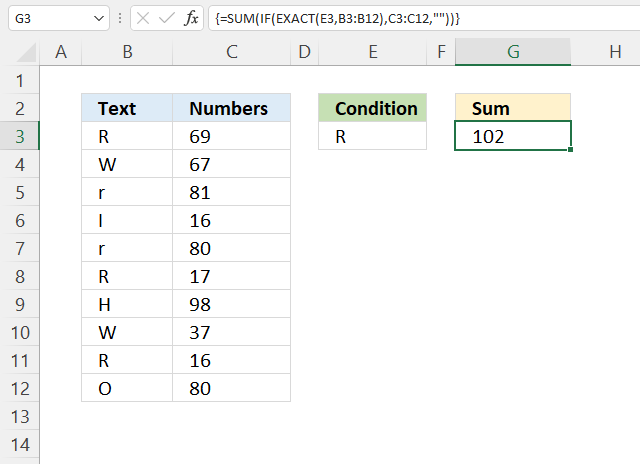Array formula in cell G3:

=AVERAGE(IF(EXACT(E3,B3:B12),C3:C12,""))

How to enter an array formula

### 6.1 Explaining formula

#### Step 1 - Compare condition to values also considering capital and lower letters

EXACT(E3, B3:B12)

becomes

EXACT("R", {"R"; "W"; "r"; "I"; "r"; "R"; "H"; "W"; "R"; "O"})

and returns

{TRUE; FALSE; FALSE; FALSE; FALSE; TRUE; FALSE; FALSE; TRUE; FALSE}.

#### Step 2 - Replace TRUE with corresponding value on the same row

The IF function returns one value if the logical test is TRUE and another value if the logical test is FALSE.

IF(logical_test, [value_if_true], [value_if_false])

IF(EXACT(E3,B3:B12), C3:C12, "")

beomes

IF({TRUE; FALSE; FALSE; FALSE; FALSE; TRUE; FALSE; FALSE; TRUE; FALSE}, C3:C12, "")

becomes

IF({TRUE; FALSE; FALSE; FALSE; FALSE; TRUE; FALSE; FALSE; TRUE; FALSE}, {69; 67; 81; 16; 80; 17; 98; 37; 16; 80}, "")

and returns

{69; ""; ""; ""; ""; 17; ""; ""; 16; ""}.

#### Step 3 - Calculate the sum

The SUM function calculates a total.

SUM(number1[number2], ...)

SUM(IF(EXACT(E3,B3:B12),C3:C12,""))

becomes

SUM({69; ""; ""; ""; ""; 17; ""; ""; 16; ""})

and returns 102. 69 + 17 + 16 = 102.

## 7. Calculate median based on a case sensitive condition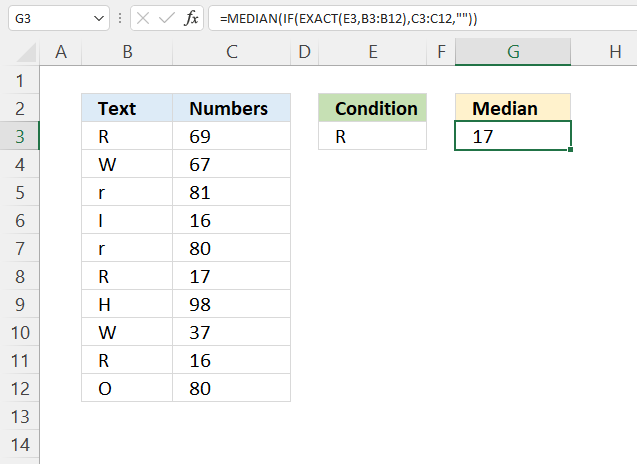Array formula in cell G3:

=MEDIAN(IF(EXACT(E3, B3:B12), C3:C12, ""))

How to enter an array formula

### 7.1 Explaining formula

#### Step 1 - Compare condition to values also considering capital and lower letters

EXACT(E3, B3:B12)

becomes

EXACT("R", {"R"; "W"; "r"; "I"; "r"; "R"; "H"; "W"; "R"; "O"})

and returns

{TRUE; FALSE; FALSE; FALSE; FALSE; TRUE; FALSE; FALSE; TRUE; FALSE}.

#### Step 2 - Replace TRUE with corresponding value on the same row

The IF function returns one value if the logical test is TRUE and another value if the logical test is FALSE.

IF(logical_test, [value_if_true], [value_if_false])

IF(EXACT(E3,B3:B12), C3:C12, "")

beomes

IF({TRUE; FALSE; FALSE; FALSE; FALSE; TRUE; FALSE; FALSE; TRUE; FALSE}, C3:C12, "")

becomes

IF({TRUE; FALSE; FALSE; FALSE; FALSE; TRUE; FALSE; FALSE; TRUE; FALSE}, {69; 67; 81; 16; 80; 17; 98; 37; 16; 80}, "")

and returns

{69; ""; ""; ""; ""; 17; ""; ""; 16; ""}.

#### Step 3 - Calculate the median

The MEDIAN function calculates the median based on a group of numbers. The median is the middle number of a group of numbers.

MEDIAN(number1, [number2], ...)

MEDIAN(IF(EXACT(E3,B3:B12),C3:C12,""))

becomes

MEDIAN({69; ""; ""; ""; ""; 17; ""; ""; 16; ""})

and returns 17.

## 8. Case sensitive condition not equal to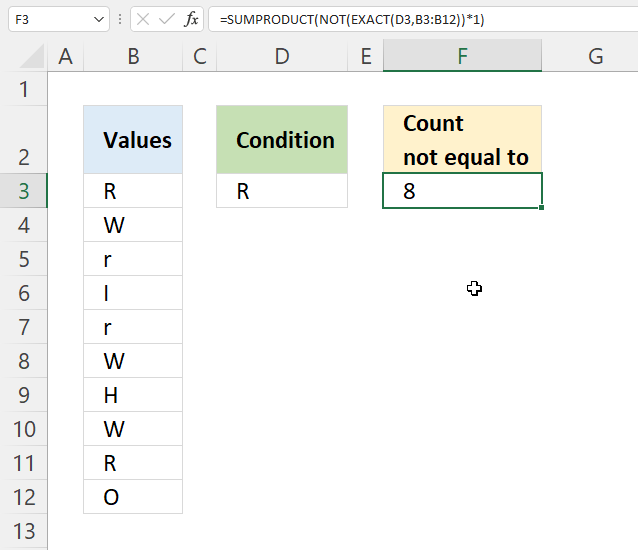The formula in cell F2 counts cells in cell range B3:B12 if not equal to the condition specified in cell D3, the count is case sensitive.

Formula in cell F3:

=SUMPRODUCT(NOT(EXACT(D3,B3,B12))*1)

### Explaining formula in cell F3

#### Step 1 - Identify cells equal to condition case sensitive

EXACT(D3,B3,B12)

becomes

EXACT("R",{"R"; "W"; "r"; "I"; "r"; "W"; "H"; "W"; "R"; "O"})

and returns

{TRUE; FALSE; FALSE; FALSE; FALSE; FALSE; FALSE; FALSE; TRUE; FALSE}.

#### Step 2 - Change TRUE to FALSE and vice versa

The NOT function returns the boolean opposite to the given argument.

NOT(EXACT(D3,B3,B12))

becomes

NOT({TRUE; FALSE; FALSE; FALSE; FALSE; FALSE; FALSE; FALSE; TRUE; FALSE})

and returns

{FALSE; TRUE; TRUE; TRUE; TRUE; TRUE; TRUE; TRUE; FALSE; TRUE}.

#### Step 3 - Multiply with 1

The SUMPRODUCT function can't add boolean values, we need to convert them to their numerical equivalents.

The asterisk character lets you multiply values in Excel formulas.

NOT(EXACT(D3,B3,B12))*1

becomes

{FALSE; TRUE; TRUE; TRUE; TRUE; TRUE; TRUE; TRUE; FALSE; TRUE}*1

and returns

{0; 1; 1; 1; 1; 1; 1; 1; 0; 1}.

#### Step 4 - Sum values

The SUMPRODUCT function adds values and returns a total, the reason we don't use the SUM function is that it requires us to enter the formula as an array formula.

SUMPRODUCT(EXACT(D3,B3,B12)*1)

becomes

SUMPRODUCT({0; 1; 1; 1; 1; 1; 1; 1; 0; 1})

and returns 8.